Visitors Online: 0 | Friday 13th December 2019CBSE Guess > Papers > Important Questions > Class XII > 2009 > Maths > Mathematics By Mr. Rajput CBSE CLASS XII
Integrate the following Q. 1.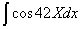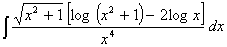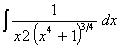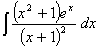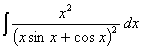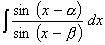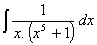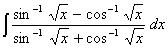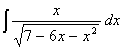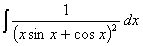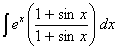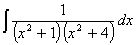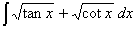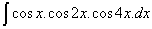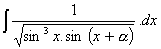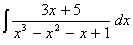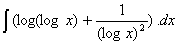Q. 2. Evaluate the following as the limit of a sum.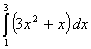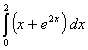Q. 3. Evaluate the following: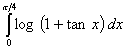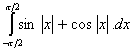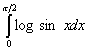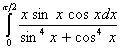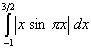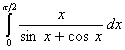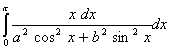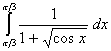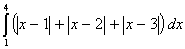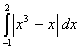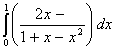Q. 4. Find the area the first quadrant enclosed by the x-axis, the line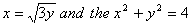Q. 5. Find the area of the region enclosed by two circles and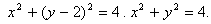Q. 6. Using integration find the area of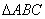, whose vertices are A(2, 0) B(4, 5) and C(6, 3). Q. 7. Find the area above x-axis and included in circle x2 + y 2 = 8x and the parabola y2 + 4x . Q. 8. Find the area of the region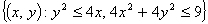Q. 9. Find the area of the circle x2 + y2 = 16 which is exterior to the parabola y2 = 6x . Differential equations Q. 1. Form the family of differential equation represented by the curve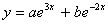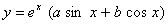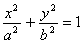Q. 2. Form the differential equation represented circle passing through origin and center lies on x-axis. Q. 3. Solve the differential equations :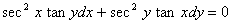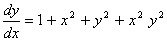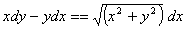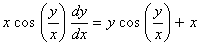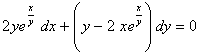Q. 4. Solve the differential equt: x(1 + y2)dx - y(1 + x2)dy = 0. y(1) = 0 (x2+y2) dx + xy dy = 0, y (1)=1 Q. 5. At any point on the curve the slope of tangent is twice the slope of the line joining the point of contact to the point(-4,-3) find the equation of the curve passing through (-2,1). Paper By Mr. Rajput